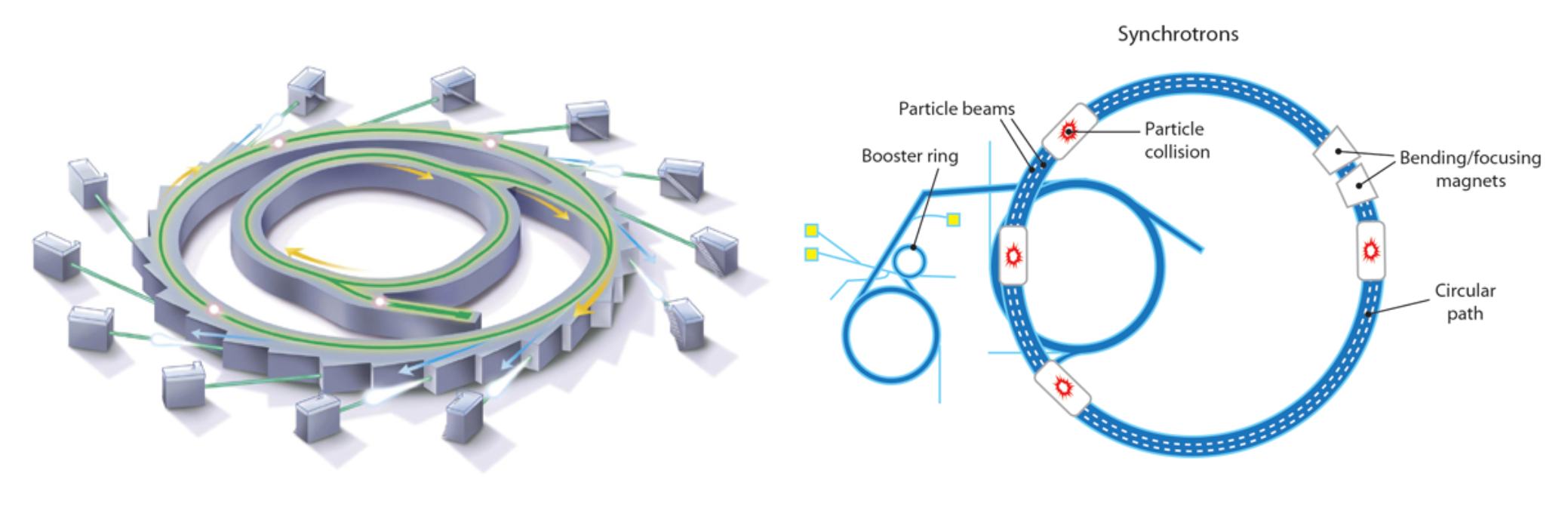# M8-S13: Particle Accelerators in Physics

### Operation of Particle Accelerators

To better understand matter, physicists examine the components of the atoms. Using particle accelerators, they separate the atom into its components by inputting large amounts of energy. This enables charged particles to accelerated to very high velocities using electric fields and/or magnetic fields.

There are three main kinds of particle accelerator:• Linear: a long track down which a particle is propelled. Particles pass through a series of tubes where alternating electrical potentials help accelerate the particle.
• Linear accelerators’ lengthy structure places limitations on the speed at which a particle can be accelerated up to. Thus, although it is simple in design, it is not used very often.• Cyclotron: a high frequency AC current is used to produce a magnetic field that causes the electron/particle to accelerate in a spiral. The charged particle is accelerated between the two semicircular regions by an electric field. This design reduces the size of the accelerator, while still using relatively simple equipment.• Synchrotron: the most modern and powerful form of particle accelerators. Synchrotrons are adapted from cyclotrons but instead consist of a close circular loop path.
• Synchrotrons accelerate particles using magnetic fields which increase in strength as a particle’s kinetic energy increases. Hence, the magnetic field synchronises with the particle.
• Synchrotrons are more effective than cyclotrons because the constantly adapting magnetic fields account for a particle’s relativistic mass (also momentum). Therefore, this set-up allows particles to reach speeds just under the speed of light.
• Magnetic fields in a synchrotron change with time such that the circular path of a particle remains constant during its acceleration. This is another distinction from cyclotrons where a particle’s path change in radius (hence the need for a disk-like structure).
• Unlike cyclotrons, synchrotrons cannot accelerate stationary particles due to their sole reliance on magnetic fields. As such, linear accelerators are typically used to produce pre-accelerated particles.
• The largest synchrotron (large hadron colliders) is 27 km in circumference.

Role of Particle Accelerators

Particle accelerators create new particles by colliding pre-existing particles head-on. The advantage of a head-on collision is that the initial total momentum is zero (or negligible) as the momenta of the particles cancel each other out. Thus, final momentum is also zero (or negligible) allowing the energy of the particles to go into a nuclear reaction. The collision of particles and their consequent splitting allows physicists to study:

• Forces between fundamental particles. Through this, it is possible to study the behaviour and range of the strong nuclear force by observing how bombarding particles are scattered when they interact with strong force.
• The nature of fundamental particles. To determine their properties (mass, structure, forces which bind them), particles are accelerated to very high velocities such that their de Broglie wavelength is short enough to probe protons/neutrons etc. This produced evidence for the Standard Model of Matter.
• The nature of matter at the time of the Big Bang – high energy situations can be simulated in particle accelerators. However, this method has not been successful in showing why the symmetry between matter and anti-matter production was broken during early stages of the Universe.
• The production of new radioisotopes when bombarding particles are absorbed.
• The energy changes which occur when particles react together.

﻿Previous section: ﻿Evidence for the Standard Model of Matter